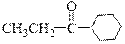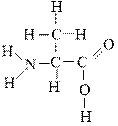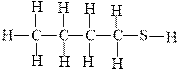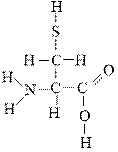# General Organic and Biological Chemistry: CHEM115 Midterm review 2 Flashcards

Set Details Share
created 7 years ago by Anbohemienne
5,521 views
3-4
Subjects:
o chem, science, chemistry
Page to share:
Embed this setcancel
COPY
code changes based on your size selection
Size:
X

1

The formula for ammonium chloride is ___.

a. AlCl3

b. NH4Cl

c. NCl

d. AmCl

2

The name of the compound Na2CO3 is

a. sodium carbonate.

b. disodium carbonate.

c. sodium bicarbonate.

d. disodium bicarbonate.

3

Helium forms an ion with a 2+ charge.

True

False

4

SO3 2- is called the sulfur trioxide ion.

True

False

5

Which is the appropriate description of Sr2+?

a. This is an atom and can be used to form chemical compounds.

b. This is an atom with a charge of +2 because there are more neutrons than protons.

c. This is an ion with a charge of +2 because there are two more protons than electrons.

d. This is an ion with a charge of +2 because there are more electrons than protons.

6

Which of the following is an anion?

a. F

b. Al3+

c. Mg

d. I-

7

Sodium carbonate is an ionic compound whose formula is NaCO3.

True

False

8

Nitrogen can have an anion, N3-. This ion

a. is the result of the atom gaining three electrons.

b. can form three ionic bonds.

c. has eight electrons in the outside orbit.

d. All of these choices are correct.

9

A compound commonly called trisodium phosphate, Na3PO4, is used to clean grease and other organic materials from concrete surfaces. Calculate the formula weight of this cleaner.

a. 70.20 amu

b. 116.70 amu

c. 118.30 amu

d. 164.00 amu

10

How many protons and electrons does Mg2+ have?

a. 12 protons and 12 electrons

b. 12 protons and 14 electrons

c. 14 protons and 12 electrons

d. 12 protons and 10 electrons

11

The name of the compound N2O4 is

a. nitrogen oxide.

b. nitrogen tetroxide.

c. dinitrogen tetroxide.

d. nitrogen dioxide.

12

What is the mass in grams of 3 moles of calcium carbonate, CaCO3, a common antacid?

a. 300 grams

b. 150 grams

c. 100 grams

d. 68 grams

13

What is the proper name of the compound N2O3?

a. nitrate

b. nitrogen(II) oxide

c. nitrogen trioxide

d. dinitrogen trioxide

14

Chose the formula of cobalt(II) nitrate.

a. CoN

b. Co2 NO3

c. Co(NO3)2

d. Co(II)(NO3)2

15

The proper name for P2O5 is

a. acetate

b. hydrogencarbonate (bicarbonate)

c. carbonate

d. cyanide

16

A Roman numeral is used in the name of transition metal ions. That numeral indicates the charge of the ion.

True

False

17

Aluminum sulfate, Al2(SO4)3, can be used in water purification. What is the formula weight of this salt?

a. 75.10 amu

b. 342.30 amu

c. 315.30 amu

d. 150.10 amu

18

A compound is

a. two or more elements well mixed.

b. two or more elements combined chemically.

c. two or more elements carrying a positive charge.

d. a physical blend of two or more elements.

19

What is the formula of beryllium sulfide?

a. BeS

b. Be2S

c. Be2S3

d. Be3S2

20

Serotonin is a molecule involved in the transmission of nerve impulses. One of the organic families present in serotonin is ___.

a. ester

b. alkyne

c. phenol

d. aldehyde

21

The correct condensed structure of Propylene glycol, a compound used as a solvent for drugs is

a. CH3CH2CH2OH

b. CH2HOHCOH2COHc.

CH3

c. CH3-CH-OH

OH

CH3

d. CH3-CH-OH

OH

22Which of the following organic families is present in the molecule provided below?

a. ether

b. ketone

c. aldehyde

d. phenol

23Which atoms in the following molecule would have δ- charge based on the electronegativities of the elements?

a. H and O

b. O and C

c. C and N

d. N and O

24

Hydrocarbons can take part in dipole-dipole interactions.

True

False

25The following molecule belongs to which family of compounds?

a. alcohols

b. Ethers

c. sulfides

d. Thiols

26

Which of the following contains a nonpolar covalent bond?

a. LiBr

b. CaCl2

c. H2O

d. O2

27

Which of the following molecules is polar?

a. H2CO (hint: C is the central atom)

b. CH4

c. N2

d. BCl(hint: B is the central atom and doesn’t have an octet)

28

The ability of an atom in a molecule to attract bonding electrons to itself is its ___.

a. polarity

b. electronegativity

c. ionic nature

d. bonding character

29

The Si-Si bond in Cl3SiSiCl3 is expected to be polar.

True

False

30

Which molecule has a linear geometry?

a. SO2

b. H2O

c. OF2

d. CO2

31

The greater the difference in electronegativity of the atoms involved, the more polar the covalent bond.

True

False

32

Organic compound must contain which of the following atoms?

a. O

b. H

. S

d. C

33

he kinds of interactions that exist between CH3COOH molecules include dipole-dipole (including hydrogen bonding) interactions and London forces

True

False

34

Which of the following statements is (are) true about hydrogen bonding interaction?

a. It occurs only in molecules that contain H bonded to a highly electronegative atom, such as N, O, or F.

b. It is a special type of dipole-dipole interaction.

c. It is present in H2O.

d. All of these choices.

35

Which pairs of molecules can form a hydrogen bond with one another?

a. NF3

b. CH3CH2CH2CH2CH3

c. CH3CH2NH2

d. CCl4

36

Which bond is least polar?

a. H-F

b. H-Cl

c. H-Br

d. H-I

37

The molecule CH4 has a tetrahedral shape. The angle between the bonds in CH4 is approximately ___.

a. 104.5°

b. 109.5°

c. 90°

d. 120°

38

Choose the type of shape from Column 2 that best matches each item in Column 1.

CO2 - Linear

NH3 pyrmidal

CH2O Tetrahedral

SO2 Bent

39Which atoms in the following molecule would have δ- charge based on the electronegativities of the elements?

a. H, C, and O

b. N, O, and S

c. C and N

d. N, S, and C

40

The name for the Ti4+ ion is

a. Tin ion.

b. Tin (IV) ion.

c. Titanium ion.

d. Titanium (IV) ion.

41

Generally, the octet rule works fairly well for predicting the behavior of atoms. However, there is an exception:

a. The halogens do not form ions on the basis of the octet rule.

b. The alkali metals only conform to the octet rule when producing neutral atoms.

c. The transition metals do not necessarily conform to the octet rule.

d. The nonmetals do not conform to the octet rule.

42

When considering the octet rule, the explanation of the O2- ion is

a. a loss of two electrons to get closer to 8 in the energy level.

b. when an oxygen atom gains two electrons, it has the same electron arrangement as neon, an inert gas.

c. oxygen’s nuclear charge of 8 can only hold 8 electrons in the outer shell.

d. oxygen is an exception to the octet rule.

43

What is the proper name of the compound FePO4?

a. iron phosphide

b. iron(III) phosphide

c. iron(III) phosphate

d. iron phosphorus tetraoxide

44

The formation of ions typically occurs when

a. nonmetal atoms lose electrons and metals gain them.

b. metal and nonmetal atoms are close together in the number of valence electrons.

c. metal and nonmetal atoms have the same number of electrons.

d. nonmetal atoms gain electrons and metal atoms lose them.

45

Which of the following is a cation?

a. Ca2+

b. Na

c. Br

d. O2-

46

Elements in family 8A tend to form anions.

True

False

47

According to the octet rule, strontium (Sr) will form which of the following monoatomic ions?

a. Sr2-

b. Sr3+

c. Sr2+

d. Sr3-

48

Predict the number of covalent bonds by N atom.

a. 1

b. 2

c. 3

d. 4

49

Which of the following is a binary compound?

a. NaOH

b. FeCl3

c. Ca 3(PO4)2

d. None of these choices is a binary compound.

50

Calculate the mass of 10.00 moles of nickel(II) cyanide, Ni(CN)2.

a. 847.0 grams

b. 987.0 grams

c. 1107 grams

d. 1694 grams

51

What is the formula of the ionic compound that forms between lithium ions and dichromate ions?

a. LiCr2O7

b. Li3 Cr2O7

c. Li2 Cr2O7

d. Li4Cr2O7

52

In which bond does the oxygen atom possess a partial positive charge?

a. O-H

b. O-F

c. N-O

d. O-C

53

Dioxygen difluoride whose actual three dimensional shape is provided below is non-polar.

True

False

54

Ethanol, C2H5OH, is found in alcoholic beverages. If a 125-g sample is pure ethanol, how many moles of ethanol are present?

a. 0.419 moles

b. 2.72 moles

c. 5750 moles

d. 46.3 moles

55

What is the correct name of the compound Cl2O3?

a. dichlorine trioxide

b. Chlorine oxide

c. Chlorine trioxide

d. Chloride oxide

56

How many valence electrons must S atom gain to reach an octet?

a. 1

b. 2

c. 3

d. 4

57

Ag+ is an ion with 47 protons and 108 electrons.

True

False

58

What is the shape around C atom in OCN-?

a. linear

b. bent

c. pyramidal

d. Trigonal planar

59

Which of the following bonds can be classified as polar?

a. NaCl

b. CH

c. CsBr

d. ClF

60

Potassium has lower electronegativity than F.

True

False

61

Which bond is most polar?

a. B-Cl

b. C-Cl

c. O-Cl

d. Cl-Br

62

The strongest noncovalent interaction that can occur between hydrocarbons is ___.

a. dipole-dipole forces

b. hydrogen bonding

c. ion-dipole interaction

d. London force

63

Which of the following is an ionic compound?

a. CS2

b. CO2

c. Mg(OH)2

d. CH4

64

Calcium chloride has the formula CaCl2. As an ionic compound it is

a. composed of one cation and 2 anions.

b. held together by means of covalent bonds.

c. composed of two cations and one anion.

d. more likely a molecular compound.

65

The formula of a phosphate ion is ___.

a. PO4 3-

b. P3-

c. HPO4 2-

d. H2PO4 -

66

How many electrons are contained in sulfide ion?

a. 18

b. 14

c. 30

. 16

67

Which symbol is representative of a halide ion?

a. He

b. Ne

c. I-

d. Fe2+

68

All molecules with polar covalent bonds are polar.

True

False